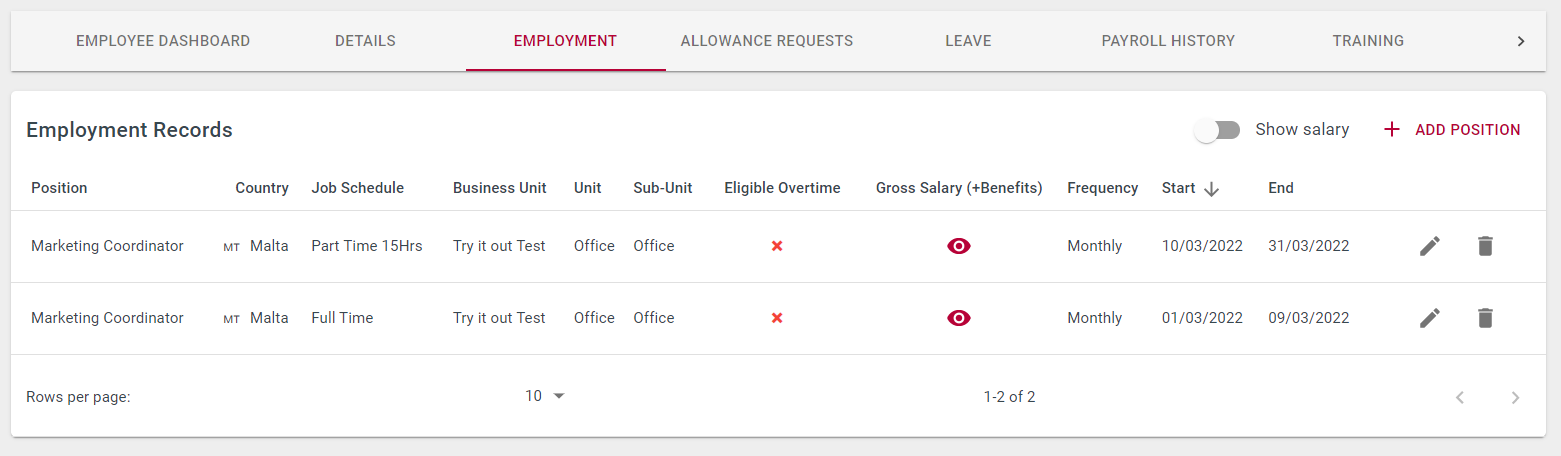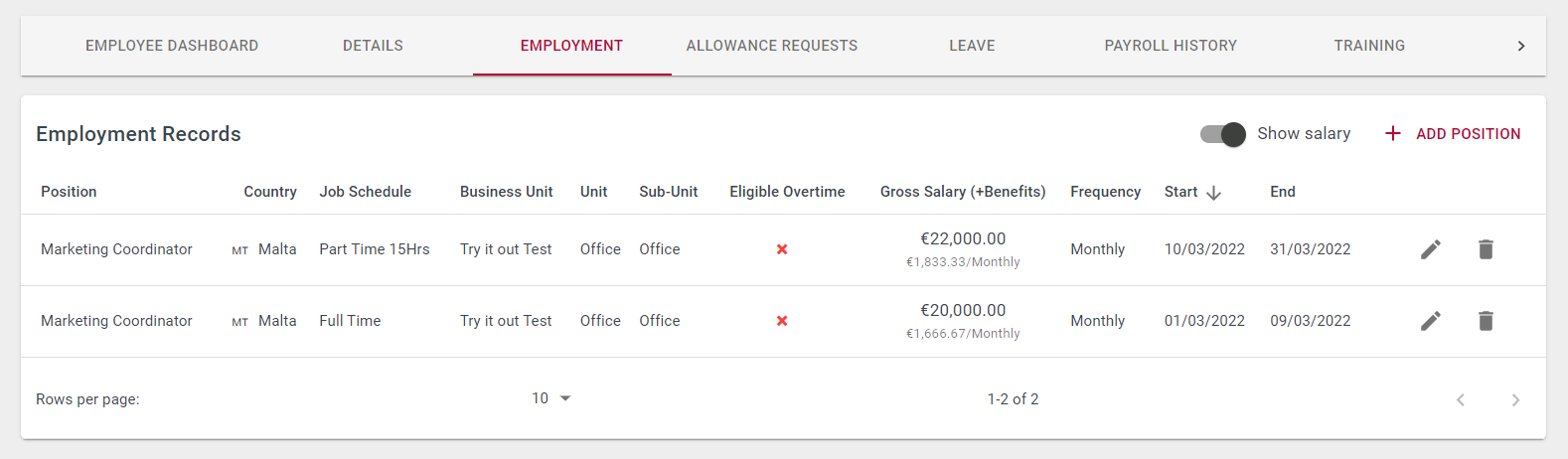# How is the Basic Pay calculated when there's a change in Position History?Talexio Support
• Updated

## Overview

When an employee changes their Job Schedule or Salary, Talexio calculates the hours/salary due for the period left in the old position and the hours/salary due for the new period.

When an employee's position is terminated, Talexio calculates the hours due for the period left in the position.

## Permissions

You need to have the Manage employment position history permission to amend an employee's position, and you need the Manage Payroll permission to work on payroll.

## Change in Job Schedule1. Calculate working days

Calculate the number of working days in each position history: from the start of payroll period till the end of position history for the old position; and from the start of position history till the end of payroll period for the new position.

Full-time: 01/03/2022 - 09/03/2022 = 7 working days
Part-time: 10/03/2022 - 31/03/2022 = 15 working days

Then calculate the number of working days in the current payroll period.
March 2022 = 22 working days

2. Calculate working hours

Calculate the working hours for each position history by multiplying the number of working days by the number of daily hours (as per job schedule).

Full-time: 7 working days * 8 hours a day = 56 working hours
Part-time: 15 working days * 4 hours a day = 60 working hours

Then calculate the total working hours for the current payroll period for both job schedules.

March 2022:
Full-time: 22 working days * 8 hours a day = 176 working hours
Part-time: 22 working days * 4 hours a day = 88 working hours

3. Calculate Basic hours to pay

Divide the working hours for each position history by the total working hours for the payroll period, then multiply by the average hours used for the payroll period.

Full-time: (56 working hours / 176 working hours) * 173.33 average monthly hours = 55.15 hours
Part-time:  (60 working hours / 88 working hours) * 86.67 average monthly hours = 59.09 hours

4. Remove unpaid hours

Deduct any hours of unpaid leave booked during any of the two positions, from the number of hours calculated in step 3.

Full-time: 55.15 hours - 8 hours unpaid leave = 55.15 hours
Part-time: 59.09  hours - 0 hours unpaid leave = 59.09 hours

5. Multiply by the hourly rate

The final number of basic hours to pay (unpaid hours deducted) must be multiplied by the hourly rate. To find the hourly rate when an employee is on an annual salary, divide it by 52 weeks, and then divide it again by the weekly working hours.

Annual salary on Full-time: €20,000 / 52 / 40
Annual salary on Part-time: €10,000 / 52 / 20
Hourly rate: €9.6154 (for both positions since there's no salary change but only job schedule change)

Full-time 47.15 hours *  €9.6154 = €453.36
Part-time: 59.09 hours * €9.6154 = €568.17

Total Basic Hours March = 106.24 (+ 8 hours unpaid)
Total Basic Pay March = €1021.53 (+ €0 unpaid leave)

## Change in Salary(examples based on a Monthly payroll with salary increase effective on the 10th March)

1. Repeat steps 1-4 above to calculate the  basic hours to pay, including the deduction of unpaid hours.

Old salary: 01/03/2022 - 09/03/2022 = 7 working days
New salary: 10/03/2022 - 31/03/2022 = 15 working days
March 2022 = 22 working days

Old salary: 7 working days * 8 hours a day = 56 working hours
New salary: 15 working days * 8 hours a day = 120 working hours
March 2022 = 176 working hours

Old salary: (56 working hours / 176 working hours) * 173.33 average monthly hours = 55.15 hours - 8 hours unpaid leave = 47.15 hours
New salary (120 working hours / 176 working hours) * 173.33 average monthly hours = 118.18 hours - 0 hours unpaid leave = 118.18 hours

2. Multiply by the hourly rate

The final number of basic hours to pay (unpaid hours deducted) must be multiplied by the hourly rate. To find the hourly rate when an employee is on an annual salary, divide it by 52 weeks, and then divide it again by the weekly working hours.

Old salary: €20,000 / 52 / 40 = € 9.6154 per hour
New salary: €24,000 / 52 / 40 = €11.5385 per hour

Old salary: 47.15  hours * €9.6154 = €453.36
New salary: 118.18 hours * €11.5385 = €1363.62

Total Basic Hours March = 165.33 (+ 8 hours unpaid)
Total Basic Pay March = €1816.98
(+ €0 unpaid leave)

Share:
<% if (previousArticle || nextArticle) { %>
<% if (previousArticle) { %>
<% if (previousTitle) { %>

#### <%= previousTitle %>

<% } %> <%= previousArticle.title %>
<% } %> <% if (nextArticle) { %>
<% if (nextTitle) { %>

#### <%= nextTitle %>

<% } %> <%= nextArticle.title %>
<% } %>
<% } %>
<% if (allItems.length > 1) { %>